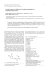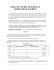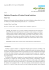# CHEM1002 Worksheet 2: Introduction to Carbon Chemistry Model 1

## Transcription

CHEM1002 Worksheet 2: Introduction to Carbon Chemistry Model 1
```CHEM1002 Worksheet 2: Introduction to Carbon Chemistry
Model 1: Bonding in Organic Molecules
Here is a partial periodic table. The shaded elements are the focus of organic chemistry. The number above
each column indicates the number of covalent bonds that an element in that column will typically make.
1
2
3
4
3 2 1
0
H
He
Li Be
B C N O F Ne
Na Mg
Al Si P S Cl Ar
K Ca … Ga Ge As Se Br Kr
Rb Sr … In Sn Sb Te I Xe
Figure 1. Partial Periodic Table
Critical thinking questions
1.
How many bonds does carbon typically make? Draw a molecule composed of only C and H with
exactly two C atoms and some number of H atoms in which both C and H are making their typical
number of bonds.
2.
Nitrogen typically forms three bonds. Given that each bond involves two electrons, and nitrogen obeys
the octet rule, how many valence electrons are unaccounted for?
3.
Free electrons, like bonding electrons, take up space. How does this lone pair of electrons affect the
shape of a molecule like NH3? (Try to draw it in 3-D.)
Sometimes atoms will form more or less than their typical number of bonds. In these cases the atoms are
said to carry a formal charge (either + or -).
4.
Here are a few examples. How does the typical number of bonds formed and the actual number of
bonds formed relate to the formal charge? Can you write this as a mathematical formula*?
O
N
H
O
H
H
H
O
O
H
H
O
O
H
H
This formula works well for heteroatoms (atoms other than C and H) but care must be taken when determining the
formal charge on C, as we will see next week.
*
5.
Determine the formal charges on the atoms indicated below.
O
O
N
H
H
H
H H
H
O
H
H
Model 2: Molecular Drawings
We saw in lectures that we can draw ethane (CH3CH3) in an abbreviated form that looks like this:
We have left off the H atoms and we have therefore not indicated where the H atoms are in space. In
fact ethane would look a little like this if we drew everything in:
Here the idea is to show the tetrahedral arrangements of groups (here just H atoms) around the
carbons. Note that we don’t draw ethane like this because it’s so seldom that we need to do anything
with the C-H bonds – they’re quite inert. But it’s important to remember that the molecule looks like
this.
Critical thinking questions
1.
Draw sketches of propane (CH3CH2CH3) which show (i) all the H atoms in 3D and (ii) only the
carbon atoms.
2.
An alkane with four carbons in a ring is called cyclobutane. Draw sketches of cyclobutane
showing (i) all the H atoms in 3D and (ii) only the carbon atoms.
Going back to the 3D diagram of ethane, above, imagine your pet chimp Biggles looked at the molecule
along the C-C bond, like this:
3.
Sketch what Biggles would see. By doing this explain what you think might be meant by the
term staggered conformation.
4.
Can you draw a conformation of ethane that might be higher in energy? What might Biggles call
this?
These drawings of molecules obtained by looking along the bonds like this are called Newman projections.
Model 3: Stick Structures
The line or stick structures shown in Models 2 and 3 are the simplest and most commonly used
representations of organic molecules. You will see these representations throughout this course and in other
units such as biochemistry and biology. As you develop your skills in drawing and reading stick structures,
always remember the 3D nature of the molecules.
When drawing stick structures, use the following set of rules:
1. Lines represent bonds
 1, 2 or 3 lines for single, double, triple bonds
2. C is the basis of the structure: organic molecules contain chains and rings of carbon atoms
 carbon atoms are not shown and are assumed to be at intersections and at the ends of lines
 C – H bonds are omitted: the number of missing H atoms can be worked out by remembering
that C will always make 4 bonds
 All heteroatoms (O, N, P, Cl etc) are shown as are H atoms bonded to them
3. When drawing neutral organic molecules, use the following valencies:
 4 for C, 3 for N, 2 for O, 1 for H, Cl, Br, I
Examples:
Compound
CH3COCH3
CH3CHClCH=CH2 or
CH3CHClCHCH2
CH3CH2CO2H or
CH3CH2COOH
Condensed structural formula
Stick representation
Critical thinking questions
1.
2.
Draw the stick representation of the following molecules:
(a)
CH3CH2CH=CH2
(b)
(CH3)2CHCH2CHO
(c)
CH2=C(CH3)CH=CH2
(d)
CH3CH2OH
(e)
CH3CHO
(f)
CH3COOH
Write down the chemical formula of each of the following molecules:
(a)
(b)
(c)
(d)
3.
The structure below is that of vitamin A. Draw its stick structure.
4.
The molecule below is lysergic acid diethyl amide. What is its chemical formula?
Key to success: practice further by completing this week’s tutorial homework
```

### 2.7 Ionic bonds are attractions between ions of opposite charge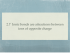### Carbon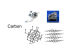### TNT is a molecule. Molecules are made up of different types of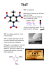### L15_Atomic-Spectra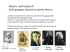### Ch. 2 Outline and Worksheet Name: Per: row: 2.1 Chemical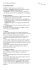### HW3 (Due, March 31, 2015) 1. The activity of a radioisotope is found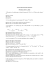### CHEM1102 Worksheet 4: Spectroscopy Workshop (1) Model 1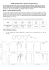### Broomfield High School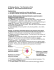### confidential great lengths price for hair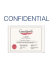### Lewis structure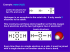### Matter, Atoms, Molecules, and Elements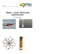### Balancing Equations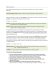### Crystal Structure of 4-Hydroxy-3-methoxybenzaldehyde-4